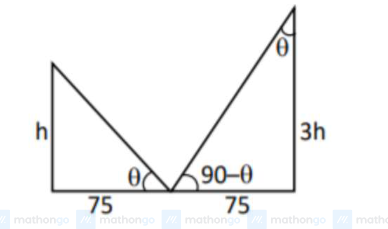# Two vertical poles are 150Question:

Two vertical poles are $150 \mathrm{~m}$ apart and the height of one is three times that of the other. If from the middle point of the line joining their feet, an observer finds the angles of elevation of their tops to be complementary, then the height of the shorter pole (in meters) is:

1. (1) 25

2. (2) $20 \sqrt{3}$

3. (3) 30

4. (4) $25 \sqrt{3}$

Correct Option: , 4

Solution:

$\tan \theta=\frac{\mathrm{h}}{75}=\frac{75}{3 \mathrm{~h}}$

$\Rightarrow \mathrm{h}^{2}=\frac{(75)^{2}}{3}$

$\mathrm{h}=25 \sqrt{3} \mathrm{~m}$